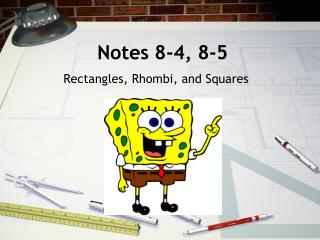DownloadDownload PresentationNotes 8-4, 8-5

# Notes 8-4, 8-5

Download Presentation## Notes 8-4, 8-5

- - - - - - - - - - - - - - - - - - - - - - - - - - - E N D - - - - - - - - - - - - - - - - - - - - - - - - - - -
##### Presentation Transcript

1. Notes 8-4, 8-5 Rectangles, Rhombi, and Squares

2. What is a Rectangle? A rectangleis a quadrilateral with four right angles.

3. Since a rectangle is a parallelogram, a rectangle “inherits” all the properties of parallelograms.

4. Example: A woodworker constructs a rectangular picture frame so that JK = 50 cm and JL = 86 cm. Find HM.

5. Example: Carpentry The rectangular gate has diagonal braces. Find HJ. HJ = GK = 48 Find HK. JG = 2JL = 2(30.8) = 61.6

6. Proving a Quadrilateral is a Rectangle in the Coordinate Plane: • Prove Definition: • Use slopes to prove opposite sides ║ (parallelogram), and consecutive sides are perpendicular (rectangle). • Prove Diagonals are congruent: • Use distance formula to prove opposite sides congruent (parallelogram), and then prove diagonals are congruent (rectangle)

7. What is a Rhombus? A rhombusis a quadrilateral with four congruent sides.

8. Example: TVWX is a rhombus. Find TV. TV =3(1.3)+ 4 = 7.9

9. Example: TVWX is a rhombus. Find mVTZ. mVTZ =[5(5) – 5)]° = 20°

10. Example: CDFG is a rhombus. Find CD. CD = 42.5 Find mGCH if mGCD = (b + 3)° and mCDF = (6b – 40)° mGCH = 17°

11. Example: Find the area of the rhombii A = ½(12)(26) = 156 Sq. Units A = ½(14)(10) = 70 Sq. Units

12. Example: Find the area of the rhombus A = 1080 m2

13. What is a Square? A square is a quadrilateral with four right angles and four congruent sides. A square is a parallelogram, a rectangle, and a rhombus.

14. To Prove a Parallelogram is a Rectangle, Rhombus or a Square: • Use distance formula to show diagonals are congruent (rectangle). • Use slopes to prove consecutive sides are perpendicular (rectangle). • Use slopes to prove diagonals are perpendicular (rhombus). • If figure is both a rectangle and rhombus, then it is a square.

15. Lesson Quiz: Part I A slab of concrete is poured with diagonal spacers. In rectangle CNRT, CN = 35 ft, and NT = 58 ft. Find each length. 1.TR2.CE 35 ft 29 ft

16. Lesson Quiz: Part II PQRS is a rhombus. Find each measure. 3.QP4. mQRP 42 51°